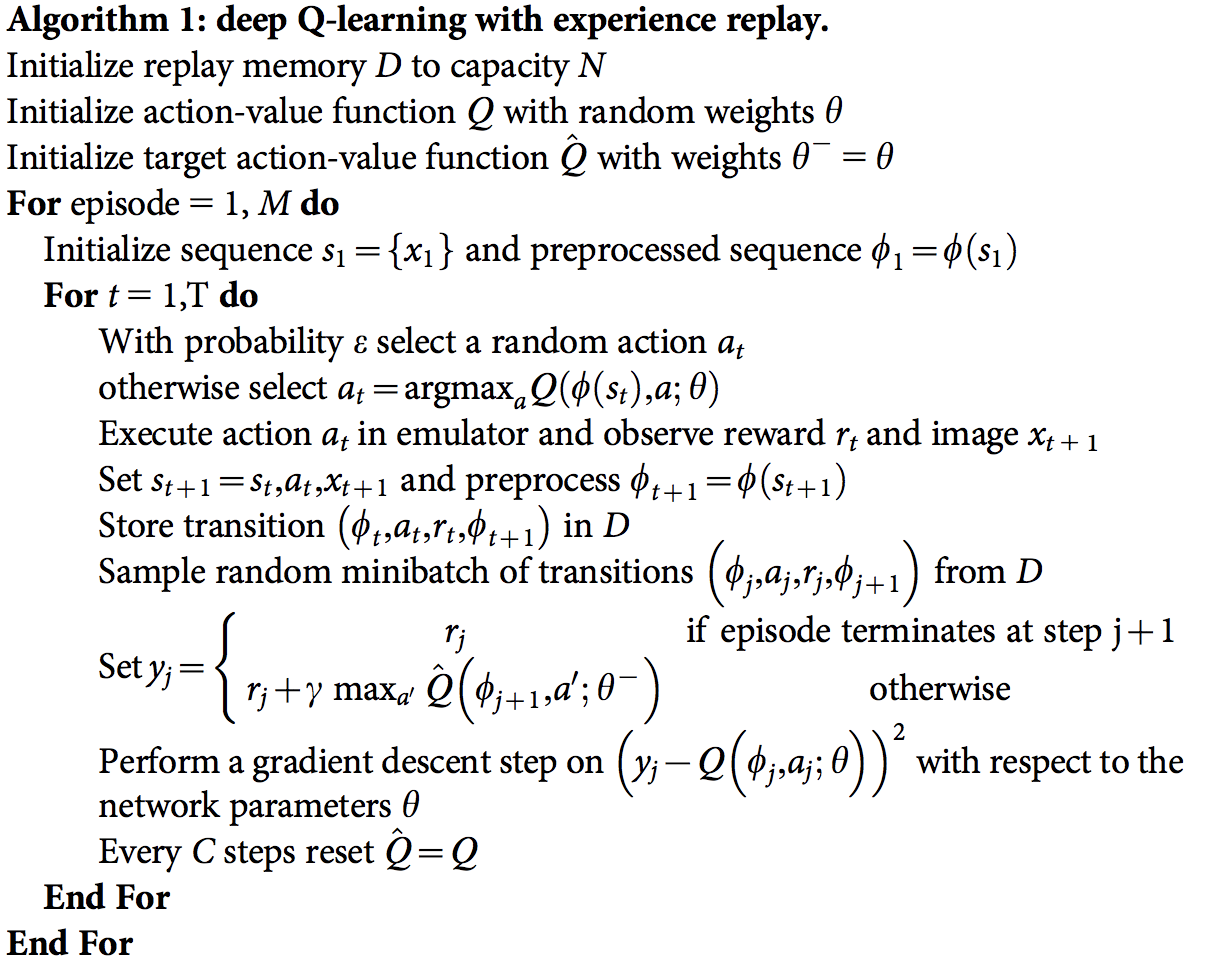# 强化学习 DQN 算法摘录以及学习资料

## 摘录

### Definations of terms

• Agent: It is an assumed entity which performs actions in an environment to gain some reward.
• Environment (e): A scenario that an agent has to face.
• Reward (R): An immediate return given to an agent when he or she performs specific action or task.
• State (s): State refers to the current situation returned by the environment.
• Policy (π): It is a strategy which applies by the agent to decide the next action based on the current state.
• Value (V): It is expected long-term return with discount, as compared to the short-term reward.
• Value Function: It specifies the value of a state that is the total amount of reward. It is an agent which should be expected beginning from that state.
• Model of the environment: This mimics the behavior of the environment. It helps you to make inferences to be made and also determine how the environment will behave.
• Model based methods: It is a method for solving reinforcement learning problems which use model-based methods.
• Q value or action value (Q): Q value is quite similar to value. The only difference between the two is that it takes an additional parameter as a current action. Q-value function gives expected total reward.
• Value-based RL: Estimate the optimal value function Q*(s,a). This is the maximum value achievable under any policy.
• Policy-based RL: Search directly for the optimal policy π∗. This is the policy achieving maximum future reward.
• Model-based RL: Build a model of the environment. Plan using model.

### Hyperparameter

1. Learning rate: The learning rate or step size determines to what extent newly acquired information overrides old information.
2. Discount factor: The discount factor γ determines the importance of future rewards.
3. Exploration factor: Exploration（探索） or exploitation（开发）.

### The process of Reinforcement Learning involves these simple steps

1. Observation of the environment
2. Deciding how to act using some strategy
3. Acting accordingly
4. Receiving a reward or penalty
5. Learning from the experiences and refining our strategy
6. Iterate until an optimal strategy is found

### Target Network

1. Since the same network is calculating the predicted value and the target value, there could be a lot of divergence between these two. So, instead of using 1one neural network for learning, we can use two.
2. We could use a separate network to estimate the target. This target network has the same architecture as the function approximator but with frozen parameters. For every C iterations (a hyperparameter), the parameters from the prediction network are copied to the target network. This leads to more stable training because it keeps the target function fixed.
3. We create two deep networks θ- and θ. We use the first one to retrieve Q values while the second one includes all updates in the training. After say 100,000 updates, we synchronize θ- with θ. The purpose is to fix the Q-value targets temporarily so we don’t have a moving target to chase. In addition, parameter changes do not impact θ- immediately and therefore even the input may not be 100% i.i.d., it will not incorrectly magnify its effect as mentioned before.

### Experience replay

1. A biologically inspired mechanism that** uses a random sample of prior actions** instead of the most recent action to proceed. This removes correlations in the observation sequence and smoothens changes in the data distribution. Iterative update adjusts Q towards target values that are only periodically updated, further reducing correlations with the target.
2. Instead of running Q-learning on state/action pairs as they occur during simulation or the actual experience, the system stores the data discovered for [state, action, reward, next_state] – in a large table.
3. For instance, we put the last million transitions (or video frames) into a buffer and sample a mini-batch of samples of size 32 from this buffer to train the deep network. This forms an input dataset which is stable enough for training. As we randomly sample from the replay buffer, the data is more independent of each other and closer to i.i.d.
4. Experience replay has the largest performance improvement in DQN.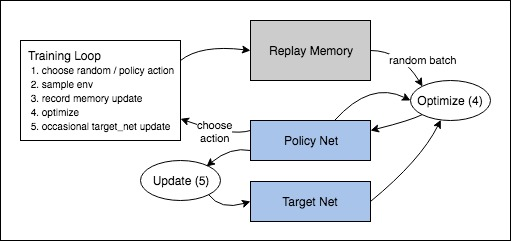## 一些思考与总结

1. 目前看到的例子中环境都是静态，离散且有限的，不清楚强化学习如何处理其他情况
2. Q-learning 的核心是 Q Table，Q Table 是一个 State-Action 表格，其中的每一项是指在当前状态下采取相应的动作可以获得的奖励
3. DQN (Deep Q-learning) leverages a Neural Network to estimate the Q-value function. In deep Q-learning, we use a neural network to approximate the Q-value function.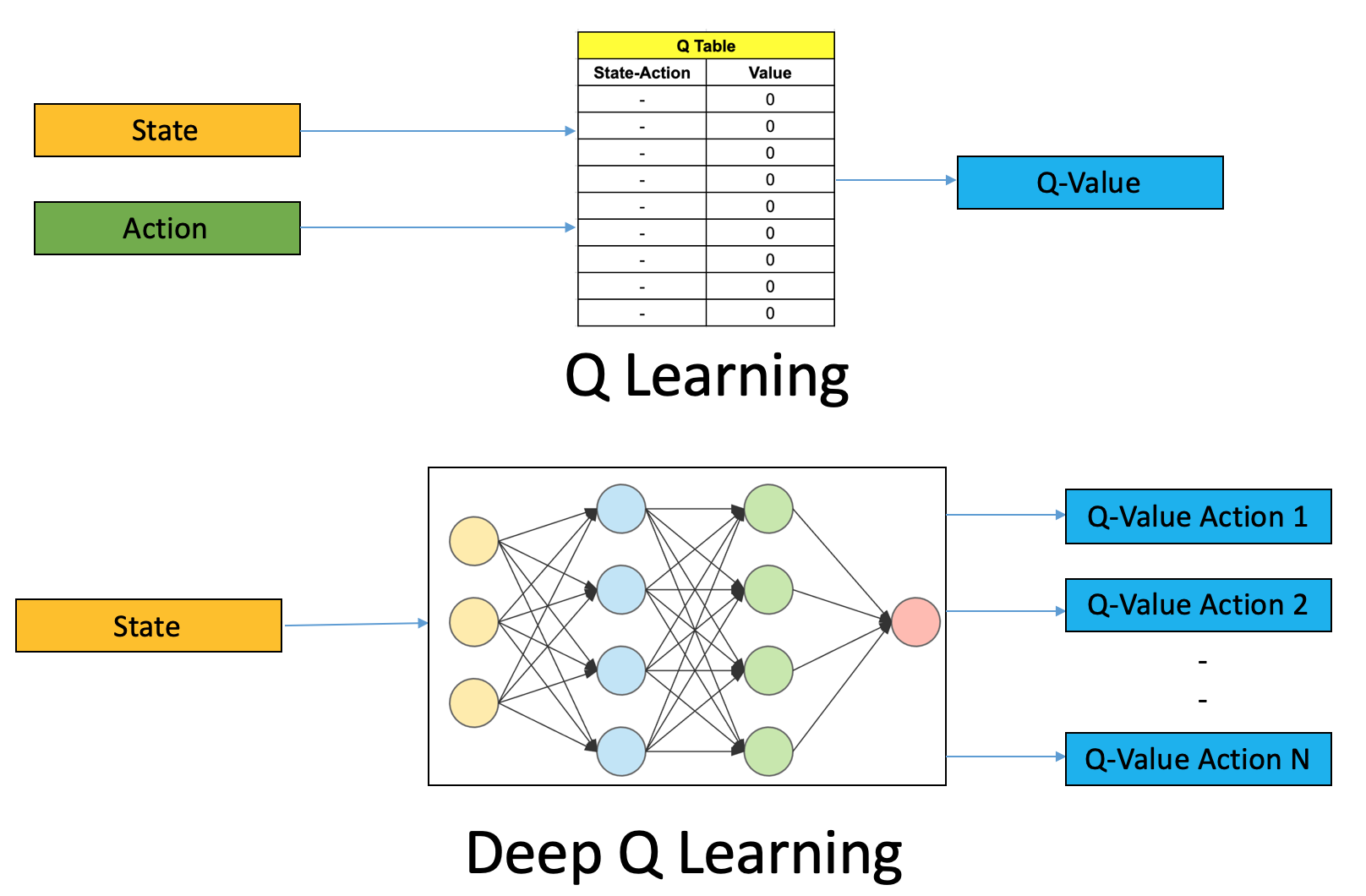4. Q 值的更新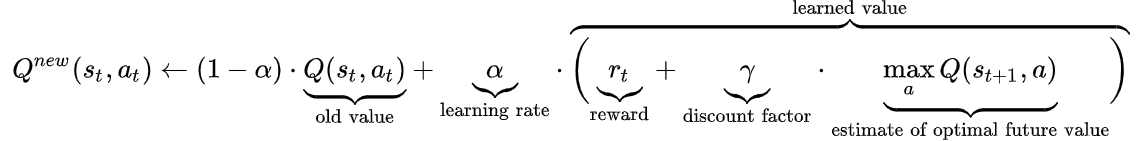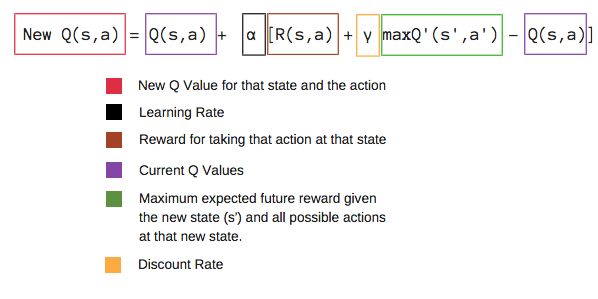5. Here are the 3 basic steps:
1. Agent starts in a state S takes an action A and receives a reward R
2. Agent selects action by referencing Q-table with highest value (max) OR by random (epsilon, ε)
3. Update q-values
6. DQN 的训练：The loss function for the network is defined as the Squared Error between target Q-value and the Q-value output from the network.
1. All the past experience is stored by the user in memory
2. The next action is determined by the maximum output of the Q-network
3. The loss function here is mean squared error of the predicted Q-value and the target Q-value – Q*. This is basically a regression problem. However, we do not know the target or actual value here as we are dealing with a reinforcement learning problem. Going back to the Q-value update equation derived fromthe Bellman equation. we have: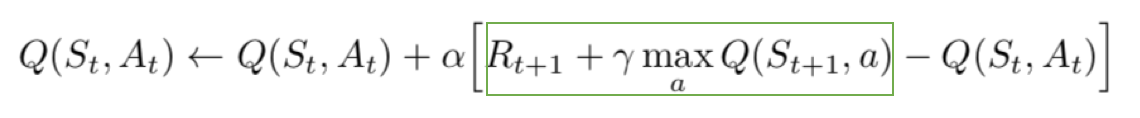The section in green represents the target. We can argue that it is predicting its own value, but since R is the unbiased true reward, the network is going to update its gradient using backpropagation to finally converge.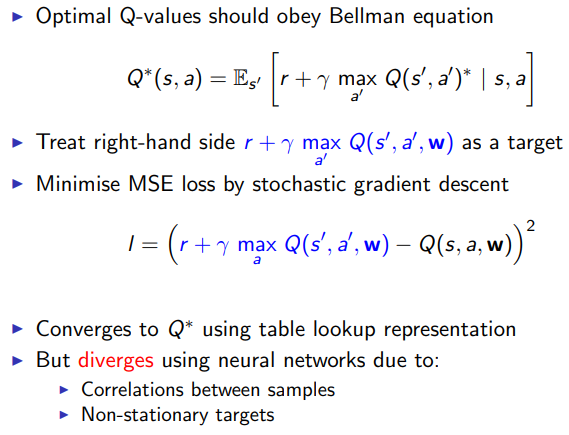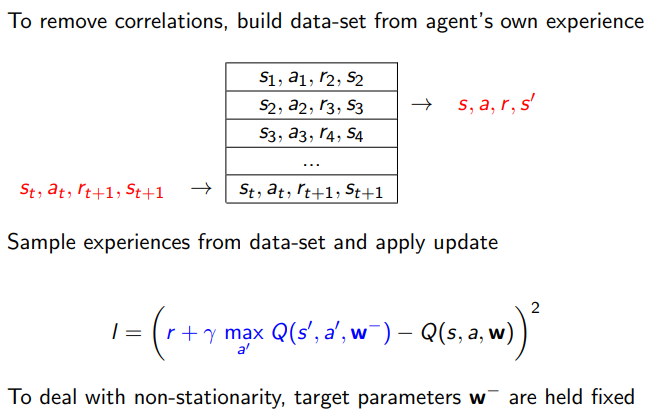``````def experience_replay(self):
if len(self.memory) < BATCH_SIZE:
return
batch = random.sample(self.memory, BATCH_SIZE)
for state, action, reward, state_next, terminal in batch:
q_update = reward
if not terminal:
q_update = (reward + GAMMA * np.amax(self.model.predict(state_next)))
q_values = self.model.predict(state)
q_values[action] = q_update
self.model.fit(state, q_values, verbose=0)
``````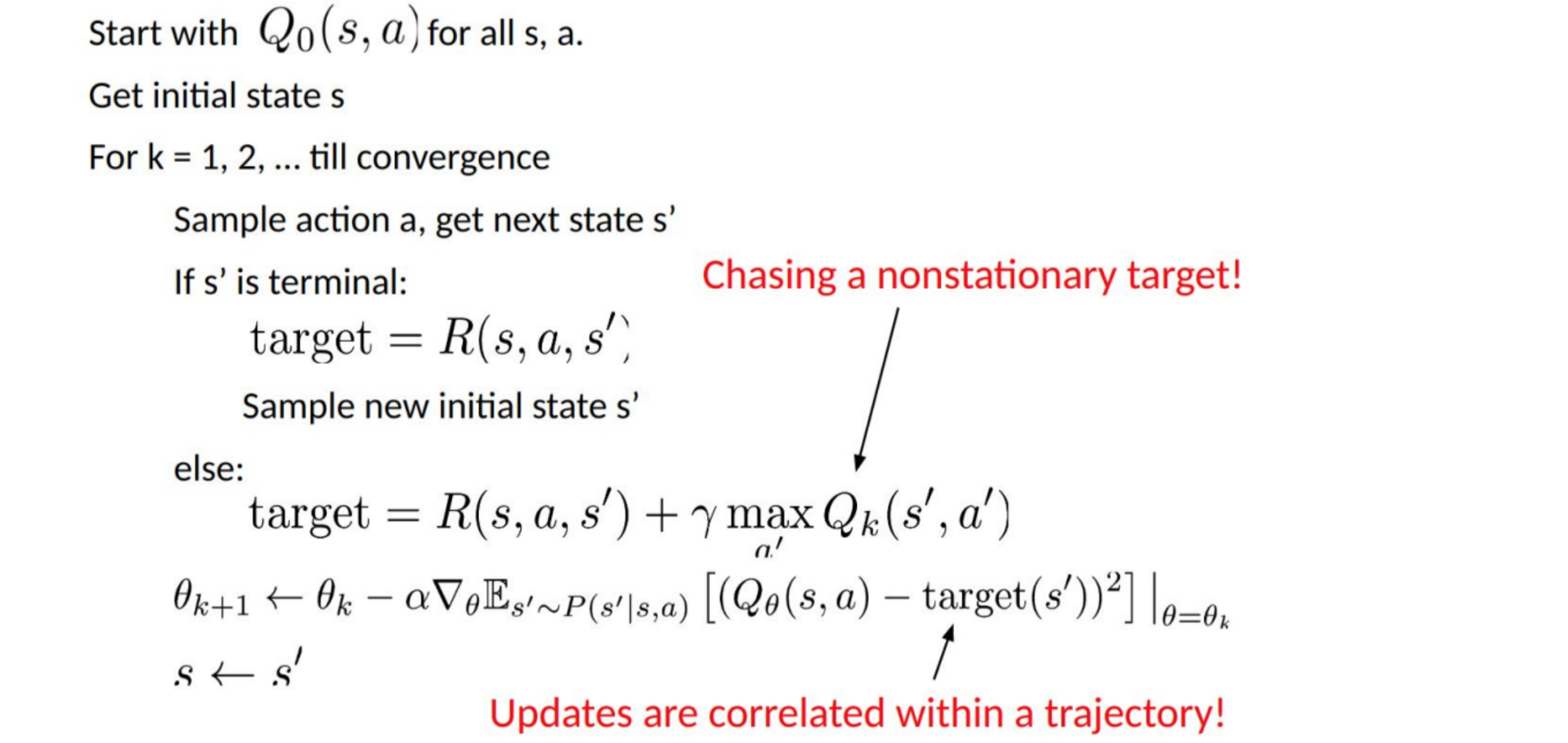1. The DQN algorithm